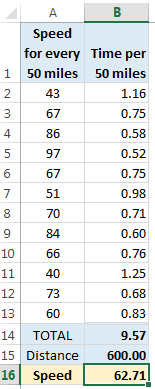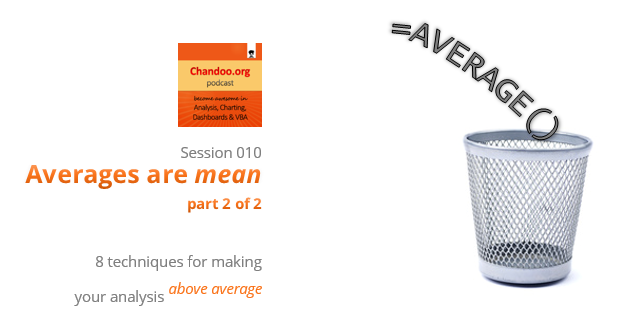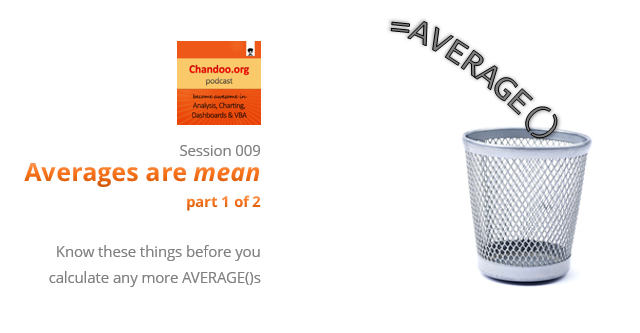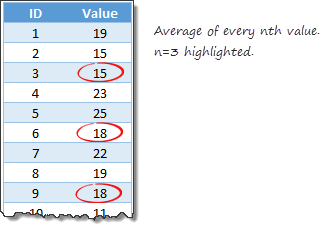# All articles with 'average()' Tag

## Weighted Average in Excel [Formulas]

Published on Sep 19, 2019 in Learn ExcelLearn how to calculate weighted averages in excel using formulas. In this article we will learn what a weighted average is and how to Excel’s SUMPRODUCT formula to calculate weighted average / weighted mean.

What is weighted average?

Wikipedia defines weighted average as, “The weighted mean is similar to an arithmetic mean …, where instead of each of the data points contributing equally to the final average, some data points contribute more than others.”

Calculating weighted averages in excel is not straight forward as there is no built-in formula. But we can use SUMPRODUCT formula to easily calculate them. Read on to find out how.

Continue »

## What is the average speed of this road trip? [Solution & Discussion]

Published on Aug 18, 2014 in Excel Howtos, Formula ForensicsLast week, we had an interesting homework problem – What is the average speed of this road trip?

We received more than 150 answers. But to my surprise, 57 of them are wrong. So today, lets learn how to calculate the average speed correct way.

Continue »

## CP010: Averages are Mean – 8 Techniques for making your analysis above average

Published on May 30, 2014 in Chandoo.org Podcast Sessions, Learn ExcelThis is a continuation of Session 9 – Averages are mean

In the earlier episode, we talked about AVERAGE and why it should be avoided. In this session, learn about 8 power analysis techniques that will lift your work above averages.

In this podcast, you will learn,

• Re-cap – Why avoid averages
• 8 Techniques for better analysis
• #2: Moving Averages
• #3: Weighted Averages
• #4: Visualize the data
• Conclusions
Continue »

## CP009: Averages are Mean – Know these things before you make any more AVERAGE()s

Published on May 22, 2014 in Chandoo.org Podcast SessionsIn the 9th session of Chandoo.org podcast, lets raise above AVERAGEs.

AVERAGEs are a very popular and universal way to summarize data. But do you know they are mean? Mean as in, AVERAGEs do not reveal much about your data or business. In episode 9 of Chandoo.org podcast, we tackle this problem and present solutions.

In this podcast, you will learn,

• What is AVERAGE?
• Pitfalls of averages
• 5 statistic concepts you must understand
• Standard Deviation
• Median
• Quartiles
• Outliers
• Distribution of data
• What next?
Continue »

## Formula Forensics No. 035 Average the last 3 values greater than 0

Published on Oct 3, 2013 in Formula Forensics, Posts by HuiLearn a technique to Average the last 3 values from a range where the values are greater than 0

Continue »

## Calculating average of every nth value [Formula tips]

Published on Sep 5, 2013 in Excel HowtosLets say you have a large list of numbers, and you want to calculate the average of every nth value. Not the average of all numbers, but just every nth number.

That is what we will learn in next few minutes.

Continue »

## Formula Forensics 016. Suzannes DJIA Average

Published on Mar 29, 2012 in Formula Forensics, Huis, Posts by Hui“I was trying to calculate the average DJIA # by month. I had the data by day so tried various combos (none of which worked) (:-”

Learn how to help Suzanne average the DJIA on a particular day and learn about a new Excel Function all in one post.

Continue »

## Find the Average of Closest 2 Numbers out of 3 [formula challenge]

Published on Jan 19, 2011 in Excel HowtosToday I am asking you a tricky formula question. This is asked by Ionel on the Introduction to VLOOKUP, OFFSET & MATCH Formulas post. The question is, I have data in three columns: A,B,C and I want to get the average of the closest two values out of three in each row. Could you help […]

Continue »

## Average of Top 5 Values [and some homework]

Published on Jun 4, 2010 in Featured, Learn ExcelThe other day, while doing consulting for one of my customers, I had a strange problem. My customer has data for several KPIs and she wants to display average of top 5 values in the dashboard. Now, if she wants average of all values, we can use AVERAGE() formula if she wants top 5 values […]

Continue »

## Calculate Moving Average

Published on Apr 28, 2009 in Learn ExcelAs part of our spreadcheats, today we will learn how to calculate moving average using excel formulas. As a bonus, you will also learn how to calculate moving average of latest 3 months alone using the count, average and offset formulas. Once you finish the tutorial, you have some home work to do as well. So go ahead and learn how to calculate moving averages.

Continue »

## Formula Debugging in Excel – Tip [spreadcheats]

Published on Dec 15, 2008 in Learn ExcelCan you imagine building a complex worksheet without formulas? I can’t. While no one can dispute the usability of formulas, we all know how painful it is when an excel formula returns a mysterious error and we don’t know what is causing the error.

Continue »

### Get FREE Excel & Power-BI Newsletter

One email per week with Excel and Power BI goodness. Join 100,000+ others and get it free.